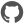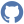# Scene

Namespace: XPlot.Plotly
Parent Module: Graph

### Constructors

 Constructor Description ``` new() ``` Signature: unit -> SceneCompiledName: `.ctor`

### Instance members

 Instance member Description ``` _isSubplotObj() ``` Signature: unit -> boolCompiledName: `set__isSubplotObj` ``` _isSubplotObj() ``` Signature: unit -> unitCompiledName: `get__isSubplotObj` ``` aspectmode() ``` Signature: unit -> objIf cube, this scene's axes are drawn as a cube, regardless of the axes' ranges. If data, this scene's axes are drawn in proportion with the axes' ranges. If manual, this scene's axes are drawn in proportion with the input of aspectratio (the default behavior if aspectratio is provided). If auto, this scene's axes are drawn using the results of data except when one axis is more than four times the size of the two others, where in that case the results of cube are used. CompiledName: `set_aspectmode` ``` aspectmode() ``` Signature: unit -> unitIf cube, this scene's axes are drawn as a cube, regardless of the axes' ranges. If data, this scene's axes are drawn in proportion with the axes' ranges. If manual, this scene's axes are drawn in proportion with the input of aspectratio (the default behavior if aspectratio is provided). If auto, this scene's axes are drawn using the results of data except when one axis is more than four times the size of the two others, where in that case the results of cube are used. CompiledName: `get_aspectmode` ``` aspectratio() ``` Signature: unit -> AspectratioCompiledName: `set_aspectratio` ``` aspectratio() ``` Signature: unit -> unitCompiledName: `get_aspectratio` ``` bgcolor() ``` Signature: unit -> stringCompiledName: `set_bgcolor` ``` bgcolor() ``` Signature: unit -> unitCompiledName: `get_bgcolor` ``` camera() ``` Signature: unit -> CameraCompiledName: `set_camera` ``` camera() ``` Signature: unit -> unitCompiledName: `get_camera` ``` domain() ``` Signature: unit -> DomainCompiledName: `set_domain` ``` domain() ``` Signature: unit -> unitCompiledName: `get_domain` ``` ShouldSerialize_isSubplotObj() ``` Signature: unit -> bool``` ShouldSerializeaspectmode() ``` Signature: unit -> bool``` ShouldSerializeaspectratio() ``` Signature: unit -> bool``` ShouldSerializebgcolor() ``` Signature: unit -> bool``` ShouldSerializecamera() ``` Signature: unit -> bool``` ShouldSerializedomain() ``` Signature: unit -> bool``` ShouldSerializexaxis() ``` Signature: unit -> bool``` ShouldSerializeyaxis() ``` Signature: unit -> bool``` ShouldSerializezaxis() ``` Signature: unit -> bool``` xaxis() ``` Signature: unit -> XaxisCompiledName: `set_xaxis` ``` xaxis() ``` Signature: unit -> unitCompiledName: `get_xaxis` ``` yaxis() ``` Signature: unit -> YaxisCompiledName: `set_yaxis` ``` yaxis() ``` Signature: unit -> unitCompiledName: `get_yaxis` ``` zaxis() ``` Signature: unit -> ZaxisCompiledName: `set_zaxis` ``` zaxis() ``` Signature: unit -> unitCompiledName: `get_zaxis`